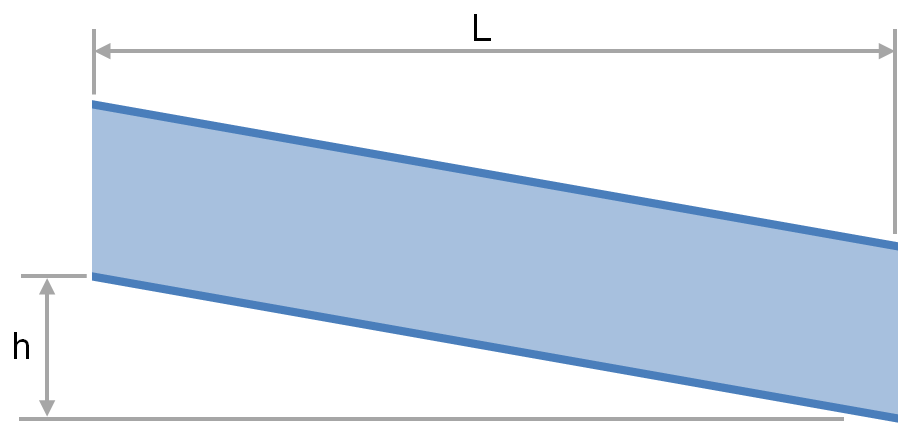f
Neutrium

# Volumetric Flow

Flow in Open Channels and Partially Filled PipesThe transport of fluid under gravity is often achieved using partially filled pipes, channels, flumes, ditches and streams. To determine the slope and elevation change required or the flow rate that is achievable one must be able to calculate the head loss and friction factor. This article provides relationships for the calculation of head loss and friction factor for fluids flowing via these conduits.

Comparing Volumetric Flow Rates for Equivalent Pressure Loss

Standard volumetric flow rates of a fluid are often used to describe the capacity of a vent or pressure relief device. To determine how this capacity compares for another fluid under different pressure and temperature conditions a conversion must be made on the basis of equivalent pressure loss. This article describes the method for calculating the volumetric flow rate of a gas which will give the equivalent pressure drop to another gas through a fixed restriction such as a vent.

Pressure Loss from Fittings in Pipe Summary

Fittings such as elbows, tees, valves and reducers represent a significant component of the pressure loss in most pipe systems. This article discusses the differences between several popular methods for determining the pressure loss through fittings. The methods discussed for fittings are: the equivalent length method, the K method (velocity head method or resistance coefficient method), the two-K method and the three-K method. In this article we also discuss method for calculating pressure loss through pipe size changes as well as control valves.

Conversion of Standard Volumetric Flow Rates of Gas

Standard volumetric flow rates of a fluid are the equivalent of actual volumetric flow rates in the sense that they have an equal mass flow rate. This identity makes standard volumetric flow appropriate providing a common baseline for comparison of volumetric gas flow rate measurements at different conditions. This article outlines how to convert between standard and actual volumetric flow rates.